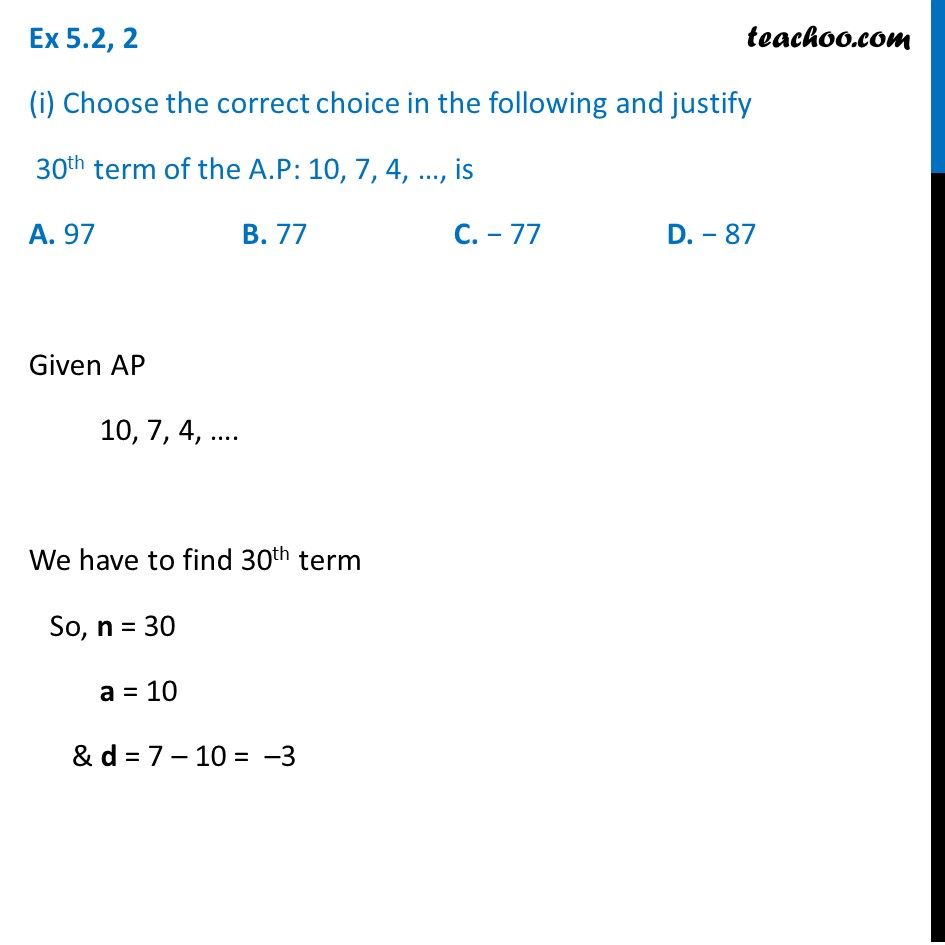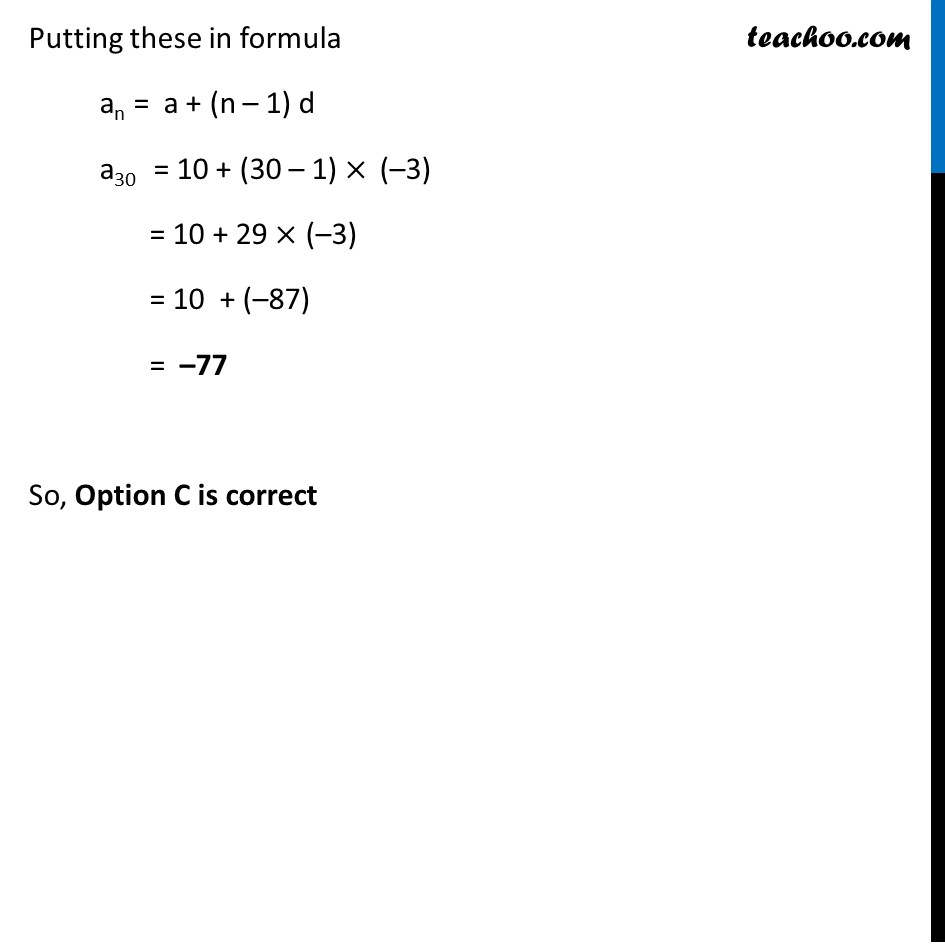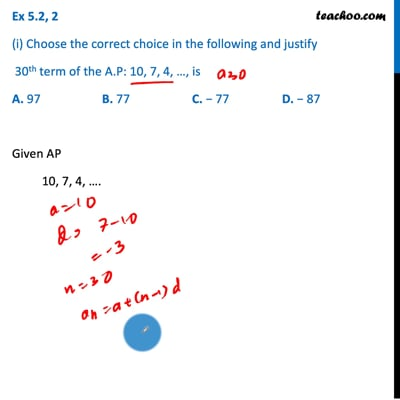Ex 5.2

Chapter 5 Class 10 Arithmetic Progressions (Term 2)
Serial order wiseThis video is only available for Teachoo black users

### Transcript

Ex 5.2, 2 (i) Choose the correct choice in the following and justify 30th term of the A.P: 10, 7, 4, …, is A. 97 B. 77 C. − 77 D. − 87 Given AP 10, 7, 4, …. We have to find 30th term So, n = 30 a = 10 & d = 7 – 10 = –3 Putting these in formula an = a + (n – 1) d a30 = 10 + (30 – 1) × (–3) = 10 + 29 × (–3) = 10 + (–87) = –77 So, Option C is correct